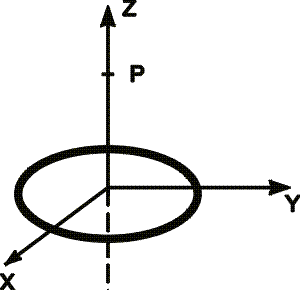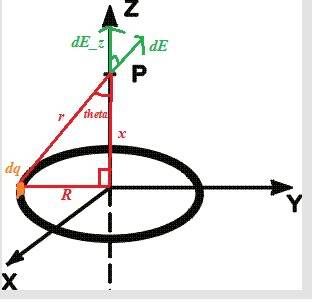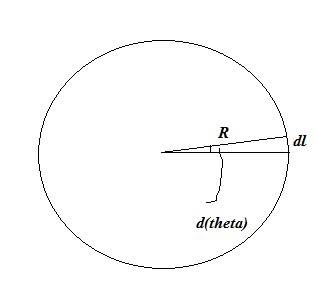# Electric field of a charged ring.

• NeverSummer

## Homework Statement

As part of the optics in a prototype scanning electron microscope, you have a uniform circular ring of charge Q=6.10 microCoulombs and radius R=1.30 cm located in the x-y plane, centered on the origin as shown in the figure.

What is the magnitude of the electric field, E at point P located at z=4.00 cm ?E=kQ/(r^2)

## The Attempt at a Solution

dE=kdQ/(r^2)=kdQ/(sqrt(h^2+r^2))=∫(k/4.206)dQ

=2.14*10^9 Q

Now, I'm not sure I've made it thus far correctly, so if I've gone wrong, please let me know. Either way, I have no idea what my limits of integration would be for this, so I'm pretty much stuck here.

I kind of follow your work, but I'm afraid I don't see the complete equation needed, so I will go ahead and derive it for you.
Alrighty, here's a little picture to help us out.Let's keep this in mind throughout the whole problem.

We know by symmetry that the electric field due to the ring at point P is going to be straight up in the Z direction. In the picture, I have the electric field element $\begin{equation} dE \end{equation}$ due to a charge element $\begin{equation} dq \end{equation}$.
Let us define that charge element. Because we have a ring, it will have a linear charge density (charge per unit length), $\lambda = Q/l = Q/2\pi R$, where L (lower case in the equation) is the circumference of the ring, it's length. Let's look at another picture for substitution reasons:This picture shows us that $\begin{equation} dl=Rd\theta \end{equation}$.
So now we can say (using the equations above) $\begin{equation} dq=\lambda dl = \frac{Q}{2\pi R} Rd\theta = \frac{Q}{2\pi} d\theta \end{equation}$

Let's not forget any of this as we move on!

We know from the equation of an electric field that $\begin{equation} dE = k \frac{dq}{r^2} \end{equation}$. Where $\begin{equation} r=\sqrt{R^2+x^2}\end{equation}$ - from the first picture.
Like said before, we know that the electric field is going to be vertically up in the Z direction, so we want to find the electric field straight up ($dE_z$) due to our charge element, we can use trig from our first picture to say this: $dE_z = dE \cos{\theta}$
And we know from our first picture again that $\cos{\theta} = R/r$

Now let's do some substitution using A LOT of what we have done before:

$dE_z = dE\cos{\theta}$

$dE_z = k \frac{dq}{r^2} \frac{x}{r}$

$dE_z = k \frac{Q/(2\pi)}{x^2+R^2} \frac{x}{\sqrt{x^2+R^2}} d\theta$

$\int dE_z = \frac{k}{2\pi} \frac{Qx}{(x^2+R^2)^{3/2}} \int_{0}^{2\pi} d\theta$ <-- integrating from 0 to 2pi (the whole ring) = 2*pi

And now we have our solution:

$E_z = \frac{kQx}{(x^2+R^2)^{3/2}}$

Now all you do is plug your numbers in

By the way this is not the only way to reach this solution, just the way I was taught. If you google "electric field due to a ring of charge" - you may find some other ways

Last edited:
Thanks for the great explanation, I got it!